# CLASS 9 SCIENCE NCERT SOLUTION FOR CHAPTER – 8 Motion

## Motion

IN TEXT Questions

Question 1.
An object has moved through a distance. Can it have zero displacement? If yes support your answer with an example.

Solution:
Yes, an object moving through a distance can have zero displacement. This happens when final position of the object coincides with its initial position. Example : If an object travels from point A and reaches to the same point A, then its displacement is zero.

Question 2.
A farmer moves along the boundary of a square field of side 10 m in 40 s. What will be the magnitude of displacement of the farmer at the end of 2 minutes 20 seconds?

Solution:
Figure ABCD is a square field of side 10 m.Question 3.
Which of the following is true for displacement?

(a) It cannot be zero.
(b) Its magnitude is greater than the distance travelled by the object.

Solution:
(a) False
(b) False

Question 4.
Distinguish between speed and velocity.

Solution:

Question 5.
Under what condition(s) is the magnitude of average velocity of an object equal to its average speed?

Solution:
The magnitude of average velocity of an object is equal to its average speed if the object moves in a straight line in a particular direction.

Question 6.
What does.the odometer of ,an automobile measure?

Solution:
The odometer of an automobile measures the distance travelled by a vehicle.

Question 7.
What does the path of an object look like when it is in uniform motion?

Solution:
In uniform motion, the path of an object can be a straight line, curved line or a circle. It can have any shape. This is because in uniform motion, speed is constant, the direction of motion may change.

Question 8.
During an experiment, a signal from a spaceship reached the ground station in five minutes. What was the distance of the spaceship from the ground station? The signal travels at the speed of light, that is 3 × 108 m s-1.

Solution:

Question 9.
When will you say a body is in

1. uniform acceleration
2. non-uniform acceleration

Solution:

1. Uniform acceleration : When a body travels with thp same velocity in the given time, then the acceleration is said to be uniform.
2. Non-uniform acceleraiion : When a body moves with unequal velocity in the equal interval of time, the body is said to be moving with non-uniform acceleration.

Question 10.
A bus decreases its speed from 80 km h’1 to 60 km h-1 in 5 s. Find the acceleration of the bus.

Solution:

Negative sign shows retardation.

Question 11.
A train starting from a railway station and moving with uniform acceleration attains a speed 40 kmh-1 in 10 minutes. Find its acceleration.

Solution:

Here, initial speed, u = 0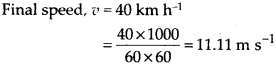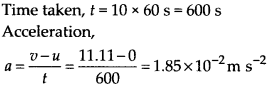Question 12.
What is the nature of the distance-time graphs for uniform and non-uniform motion of an object?

Solution:
When the motion is uniform, the distance-time graph is a straight line with some slops. When the motion is non-uniform, the distance time graph is not a straight line.

Question 13.
What can you say about the motion of an object whose distance-time graph is a straight line parallel to the time axis?

Solution:
When distance-time graph of an object is a straight line parallel to the time axis, distance of the object at every instant of time is the same. Therefore, the object must be at rest.

Question 14.
What can you say about the motion of an object if its speed-time graph is a straight line parallel to the time axis?

Solution:
When speed-time graph of a body is a straight line parallel to the time axis, speed of the body at every instant of time is the same. It means the body is moving with a uniform speed. There is no acceleration at all.

Question 15.
What is the quantity which is measured by the area occupied below the velocity-time graph ?

Solution:
Area occupied below the velocity-time graph is a measure of the distance travelled by the body or the displacement of the body.

Question 16.
A bus starting from rest moves with a uniform acceleration of 0.1 m s-2 for 2 minutes. Find

(a) the speed acquired,
(b) the distance travelled.

Solution:

Question 17.
A train is travelling at a speed of 90 km h-1. Brakes are applied so as to produce a uniform acceleration of – 0.5 m s-2. Find how far the train will go before it is brought to rest.

Solution:

Here, initial speed,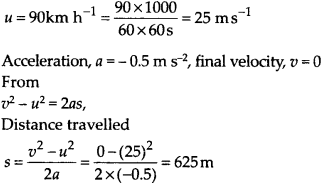Question 18.
A trolley, while going down an inclined plane, has an acceleration of 2 cm/s2 starting from rest, what will be its velocity 3 s after the start?

Solution:Question 19.
A racing car has a uniform acceleration of 4 m s’2. What distance will it cover in 10 s after start?

Solution:
Here, a = 4 m s-2, t = 10 s, u = O.The distance covered in 10 s by the car is 200 m.

Question 20.
A stone is thrown in vertically upward direction with a velocity of 5 m s-1. If the acceleration of the stone during its motion is 10 m s-2 in the downward direction, what will be the height attained by the stone and how much time will it take to reach there?

Solution:
u = 5 m s-1, v = 0, a = -10 m s-2

## Exercises

Question 1.
An athlete completes one round of a circular track of diameter 200 m in 40 s. What will be the distance covered and the displacement at the end of 2 minutes 20 s?

Solution:

Displacement after 3.5 rounds = diameter of the track = 200 m

Question 2.
Joseph jogs from one end A to the other end B of a straight 300 m road in 2 minutes 30 seconds and then turns around and jogs 100 m back to point C in another 1 minute. What are Joseph’s average speeds and velocities in jogging, (a) from A to B and (B) from A to C?

Solution:

(a) From A to B.(b) From A to C.
Time taken = A to B + B to C, 150 + 60 = 210 s Total distance = 300 + 100 = 400 mQuestion 3.
Abdul, while driving to school, computes the average speed for his trip to be 20 km h-1. On his return trip along the same route, there is less traffic and the average speed is 30 km h-1 What is the average speed for Abdul’s trip?

Solution:
Let the school be at a distance of x km. If t is time taken to reach the school, then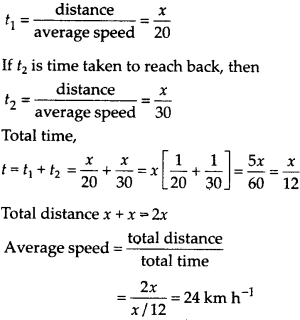Question 4.
A motorboat starting from rest on a lake accelerates in a straight tine at a constant rate of 3.0 m s-2 for 8.0 s. How far does the boat travel during this time?

Solution: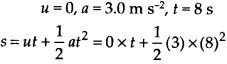Question 5.
A driver of a car travelling at 52 km h-1 applies the brakes and accelerates uniformly in the opposite direction. The car stops in 5 s. Another driver going at 3 km h-1 in another car applies his brakes slowly and stops in 10 s. On the same graph paper, plot the speed versus time graphs for the two cars. Which of the two cars travelled farther after the brakes were applied?

Solution:
The data given in this numerical problem are in different units. So, we should first convert km h-1 unit into m s-1 unit.
For first car: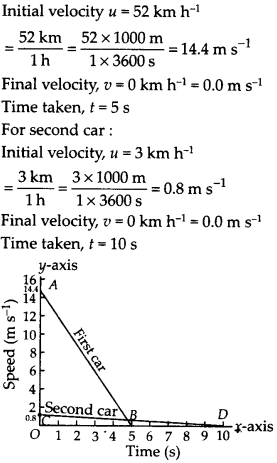The distance traveled by a moving body is given by the area under its speed-time graph. so, Distance traveled by the first car=Area of the triangle AOB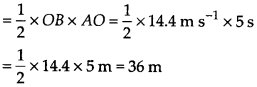Similarly, Distance travelled by the second car = Area of triangle COD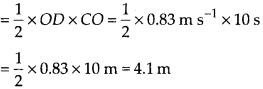Thus, the second car travels 4.1 m and the first car travels 36 m before coming to rest. So, the second car traveled farther after the brakes were applied.

Question 6.
Figure given below shows the distance-time graph of three objects A, B and C. Study the graph and answer the following questions?(a) Which of the three is travelling the fastest?
(b) Are all three ever at the same point on the road?
(c) How far has C travelled when B passes A?
(d) How far has B travelled by the time it passes C?

Solution:

(a) B is travelling fastest.

(b) As three lines do not meet at any point, the three objects never meet on the road.

(c) B passes A at D. At this time, C is at E, which corresponds to 7 km. Hence when B crosses A, then C is at 7 km from the origin.

(d) By the time B passes C, it has travelled 4.5 km.

Question 7.
A ball is gently dropped from a height of 20 m. If its velocity increases uniformly at the rate of 10 m s-2, with what velocity will it strike the ground? After what time will it strike the ground?

Solution: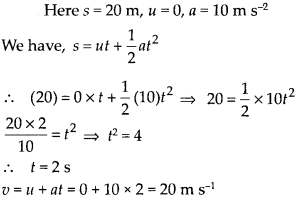The ball strike the ground after 2 s with the velocity of 20 m s-1.

Question 8.
The speed-time graph for a car is shown in the figure.(a) Find how far does the car travel in the first 4 seconds. Shade the area on the graph that represents the distance travelled by the car during the period.
(b) Which part of the graph represents uniform motion of the car?

Solution:
The motion during first 4 seconds is not uniformly accelerated. So, distance travelled by car in first 4 seconds is calculated by graphical method.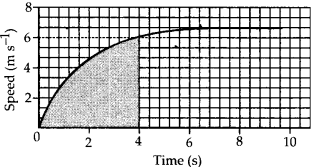(a) Number of squares in shaded part of the graph = 320.5 one small square in x axis represent t =$\frac { 2 }{ 5 } s$(b) The limiting flat portion of the curve describes the constant speed of the car, i.e., a speed of 6.0 m s-1. At this stage, the acceleration of the car is zero.
Therefore, portion of the graph between t = 6 s to 10 s, describes the uniform motion of the car.

Question 9.
State which of the following situations are possible and give an example for each of these:

(a) an object with a constant acceleration but with zero velocity
(b) an object moving in a certain direction with an acceleration in the perpendicular direction.

Solution:
(a) Free fall of an object.
(b) Object moving in a circular path

Question 10.
An artificial satellite is moving in a circular orbit of radius 42250 km. Calculate its speed if it takes 24 hours to revolve around the earth.

Solution:
Radius of the orbit = 42250 km
= 42250 × 1000 m Time taken for one revolution = 24 hours
= 24 × 60 × 60 s